## Example Questions

### Example Question #71 : Solid Geometry

The volume of a 6-foot-tall square pyramid is 8 cubic feet. How long are the sides of the base?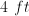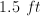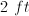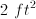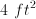Explanation:

Volume of a pyramid is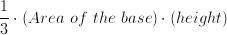Thus: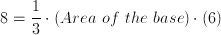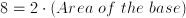Area of the base is.

Therefore, each side is.

### Example Question #1 : Pyramids

A right pyramid with a square base has a height that is twice the length of one edge of the base.  If the height of the pyramid is 6 meters, find the volume of the pyramid.

12

18

6

24

30

18

Explanation:

If the height, which is twice the length of the base edges, measures 6 meters, then each base edge must measure 3 meters.

Since the base is a square, the area of the base is 3 x 3 = 9.

Therefore the volume of the right pyramid is V = (1/3) x area of the base x height = 1/3(9)(6) = 18.

### Example Question #2 : How To Find The Volume Of A Pyramid

Find the volume of the pyramid shown below: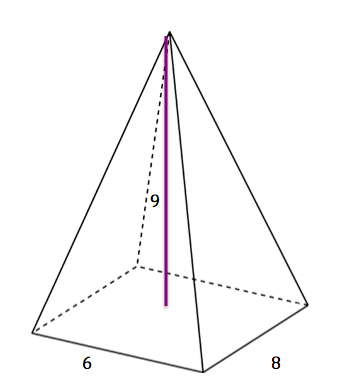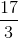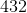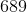Explanation:

The formula for the area of a pyramid is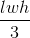. In this case, the length is, the width is, and the height is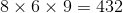and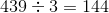### Example Question #3 : How To Find The Volume Of A Pyramid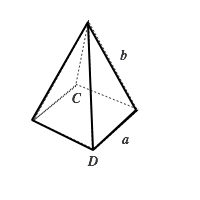Figure not drawn to scale

In the pyramid above, the base is a square. The distance between points C and D is 6 inches and the length of side b is 5 inches. What is the volume of this pyramid?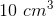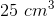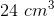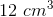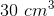Explanation: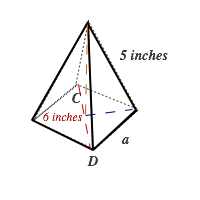To find the volume of a pyramid, you need to use the equation below: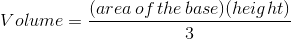To find the height (shown by the yellow line), we can draw a right triangle using the yellow line, blue line and side b (5 inches). Because the hypotenuse is 5 inches, using the common Pythagorean 3-4-5  triple. The blue line is 3 inches and the yellow line (height) is 4 inches. Also, to find side a, we can use the blue line (3 inches) and half of the red line (3 inches)  and the Pythagorean Theorum.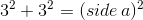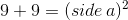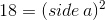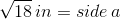Because the base is a square, the area of the base is equal to the square of side a: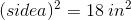Now we plug in these values to find the volume: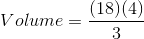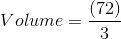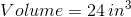### Example Question #4 : How To Find The Volume Of A Pyramid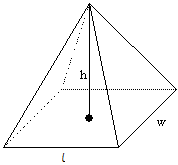Calculate the volume of the rectangular pyramid with height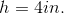, base width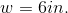, and base length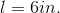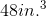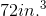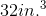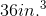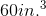Explanation:

The volumeof a rectangular pyramid with height, base width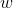, and base lengthis given by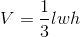.

For this pyramid,, andTo calculate its volume, substitute the values for, andinto the formula: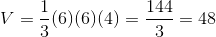Therefore, the volume of the given rectangular pyramid is